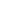# A number when divided by 312 leaves 112 as remainder. When the same number is divided by 52, the remainder will be:

a) 8
b) 18
c) 28
d) 38

 8. Solution Let x = 312y + 112 = (52 x 6y + 52 x 2) + 8 = 52 (6y + 2) + 8 Thus, when the number is divided by 52, the remainder is 8.
Share :
+
Next
« Prev
Prev
Next »